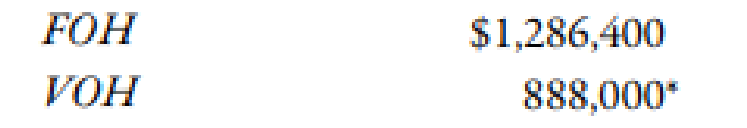Chapter 10, Problem 74P### Managerial Accounting: The Corners...

7th Edition
Maryanne M. Mowen + 2 others
ISBN: 9781337115773

#### Solutions

Chapter
Section### Managerial Accounting: The Corners...

7th Edition
Maryanne M. Mowen + 2 others
ISBN: 9781337115773
Textbook Problem
5 views

# Extrim Company produces monitors. Extrim’s plant in San Antonio uses a standard costing system. The standard costing system relies on direct labor hours to assign overhead costs to production. The direct labor standard indicates that 4 direct labor hours should be used for every monitor produced. (The San Antonio plant produces only one model.) The normal production volume is 120,000 units. The budgeted overhead for the coming year is as follows:*At normal volume.Extrim applies overhead on the basis of direct labor hours.  During the year, Extrim produced 119,000 units, worked 487,900 direct labor hours, and incurred actual fixed overhead costs of $1.3 million and actual variable overhead costs of$927,010.Required: 1. Calculate the standard fixed overhead rate and the standard variable overhead rate. 2. Compute the applied fixed overhead and the applied variable overhead. What is the total fixed overhead variance? Total variable overhead variance? 3. CONCEPTUAL CONNECTION Break down the total fixed overhead variance into a spending variance and a volume variance. Discuss the significance of each. 4. CONCEPTUAL CONNECTION Compute the variable overhead spending and efficiency variances. Discuss the significance of each.

1.

To determine

Compute the value of standard fixed overhead rate and standard variable rate.

Explanation

The amount obtained when actual overhead is deducted from applied overhead is known as overhead variance. Overhead variance is calculated to find whether the overhead is over applied or under applied.

Use the following formula to calculate the fixed overhead rate:

Substitute $1,286,400 for fixed overhead cost, 120,000 units for units produced and 4 for direct labor hours in the above formula. Fixed Overhead Rate=$1,286,400120,000×4hours=$2.68 per direct labor hour Therefore, the fixed overhead rate is$2

2.

To determine

Calculate the value of applied fixed overhead and applied variable overhead. Also, calculate the value of total fixed overhead variance.

3.

To determine

Divide the total fixed overhead variance into a spending variance and a volume variance. Also, explain the significance of each variance.

4.

To determine

Calculate the value of variable overhead spending and efficiency variances. Also, explain the significance of each variance.

### Still sussing out bartleby?

Check out a sample textbook solution.

See a sample solution

#### The Solution to Your Study Problems

Bartleby provides explanations to thousands of textbook problems written by our experts, many with advanced degrees!

Get Started

#### Find more solutions based on key concepts